5G - Massive MIMO                                                          Home : www.sharetechnote.com

Massive MIMO Channel Model

There can be many different variations of implementing the Massive MIMO and with different variation we will have different channel model. It would not be possible to post all the possible channel model here. I will just put some typical examples just to let you have chance to get familiar with various notations and mathematical formula. So don't try to memorize it and follow the equation line by line and see if those equations make sense to you. One good way is to try to copy the equation/formula on a sheet of paper by hand. This is what I am doing ..  When I am writing this page, I usually write down on a sheet of paper key equations from the document and then type in the comments onto power point and add comments around the equation based on my understanding. Just repeating this process, I found myself gradually getting familiar to basic concepts of the Massive MIMO channel model. (If you are completely new to the concept of channel model itself, I recommend to go through 'Channel Model' page (this is for generic modeling, not for MassiveMIMO. But it will help you to get familiar with basic concepts of channel model and formulating it in mathematical expression)

What kind of situation is considered in the channel model ?

There are many different situations/factors in radio channel we have to model, but there is no single model that can represents all the different situations. So the practical approach is to define several typical situations and derive different channel model (mathematical model) for each of those typical cases. In reality, it is highly likely that BTS and UE implements algorithms for all of these situations and select one of them depending on the specific situation (this is similar concept in current LTE transmission model (TM) design).

In this section, I will introduce the technical components of Massive MIMO channel model described in METIS documents (Ref )). I strongly recommend you to read the METIS document first if you are specifically interested in this area. In this section, I will just blindly copy the illulstration from the METIS document, but will try to put comments in my own words. Go through each cases several times whenever you have chance until you get clear picture of each cases and then try to read technical papers and try to associate each of the papers you read to one of the system models introduced here. In most technical papers, they don't usually illustrate the big pictures and jump into the details right away. So if you can correlate one of these big pictures to the papers you read, it will be very helpful for you to understand the contents.

 Technology Component 1 : Communicating in the near field of a large aperture massive array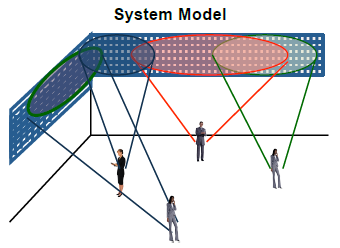This model shows a case where (usually) a single cell with huge array of antenna and it covers multiple users simultaneously. So there can be high possibility of interference with signals for different users. However, we don't consider interference by the signal from neighbouring cells. This might be the case where we can achieve the maximum throughput. In this case, you may need to consider following factors into the channel model. MU MIMO (Multi-User MIMO) BeamForming Intracell Interference

 Technology Component 2 : Low rate and simple coordination techniques for pilots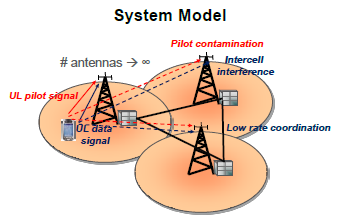This is the low data rate situation but in dense network. In this case, UE will experience high interference from neibouring cell and Network will experience interference from UEs under the coverage of other cells. In this scheme, Network tries to mitigate this interference (especially Pilot contamination) problem by coordinating with neighbouring networks.

 Technology Component 3 : Modelling with spherical waves, Linear precoding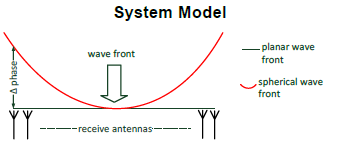In most of the channel model, we assume wavefront reaching the receiver antenna is plannar, which means that the distance between each of Tx antenna in the array and the reciever antenna is same or the differences differ in multiples of wavelength. If the number of Tx antenna in the array is not big, this assumption would be valid. However, if the number of Tx antenna in the array is huge, the difference between each of Tx antenna and the reciever antenna would not be negligiable. In this case, we have to assume that the wavefront reaching the Rx antenna would be spherical. This channel model should consider the spherical wavefront into account.

 Technology Component 4 : Multicell MU-MIMO in real world scenarios-antenna calibration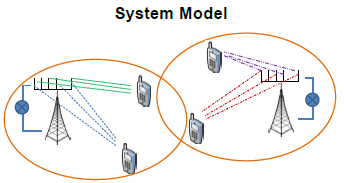In massive MIMO, it is important and challenging to calibrate each of the antenna ports (If Calibration is not done properly, the result of beamforming would not reach the intended UE). In this model, a calibration procedure with help of UE will be investigated.   Following factors will be taken into account into this model. Precoding Scheme Link Adaptation and Interference in CQI report

 Technology Component 5 : Model based channel prediction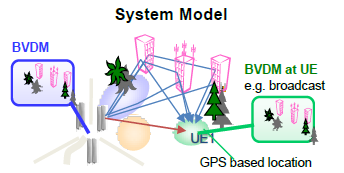Technology Component 6 : Robust and energy efficient wireless backhaul for very fast moving relays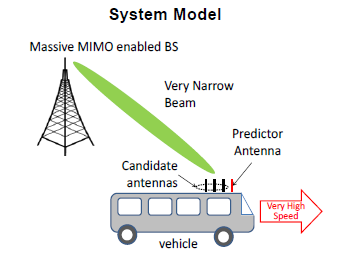Technology Component 7 : Massive MIMO Transmission Using Higher Frequency Bands Based on Measured Channels with CSI Error and Hardware Impairments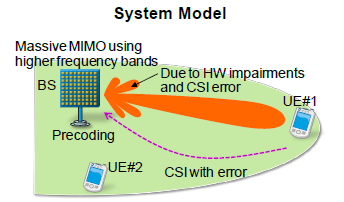When applying the MassiveMIMO (a lot of downlink antenna), one of the biggest challenge would be to estimate the quality of the downlink channel quality. It would be difficult to use downlink refernece signal method as we are using in current LTE since it will require too much resource allocation for reference signal. How to handle this situation would be an important topics in 5G channel model.

 Technology Component 8 : Integrating a Massive-MIMO macro network with a dense layer of small cells (SCs)�two tier deployment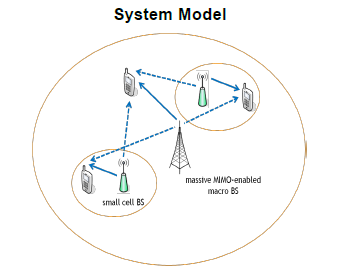Technology Component 9 : Multi-cell MU MIMO maximizing weighted sum rateWhen a network is communicating simultaneously with multiple users in a cell, the signal would various types of interference from other UEs within the same cell and interference from neighbouring cells. We need to figure out the best configuration (Tx power, Resource Allocation) for each UEs to maximize the total throughput of a cell.

 Technology Component 10 : Multi-cell MU MIMO, decentralized transceiver design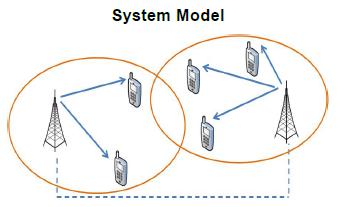Technology Component 11 : Heterogeneous Multi-cell, MU Massive-MIMO, massive SDMA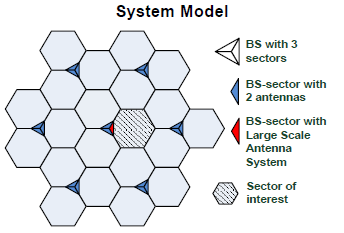Massive MIMO Channel Model - Examples

At first, I tried to put all the examples here but I realized that it is getting longer faster than I thought and it would slow down the page loading. So I decided to create separate pages (notes) for each example and put the link here.

The titles of each example is same as the title of the original title of the paper or thesis. If you click the title, it would show up my notes for it.

There is no technical importance/implication in terms of the order of the example. It just happened that I read those papers/thesis in that order.

1. Channel Model for Point to Point MIMO

2. Channel Model for Multi User MIMO

3. Channel Model : Massive MIMO and Small Cells

4. Channel Model : FD(Full Dimension) MIMO/3D MIMO

Reference